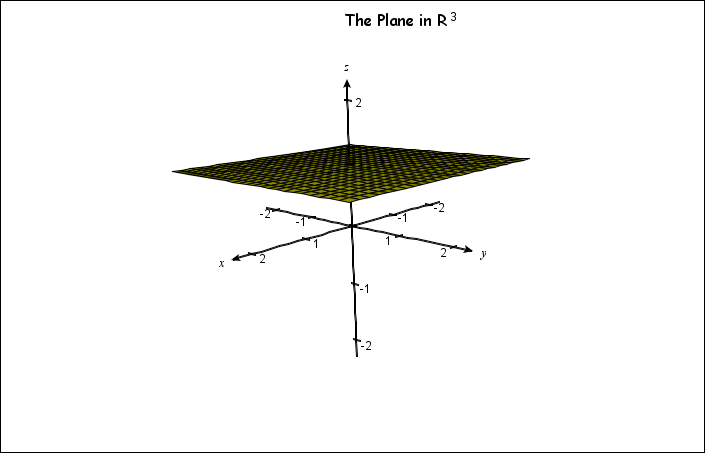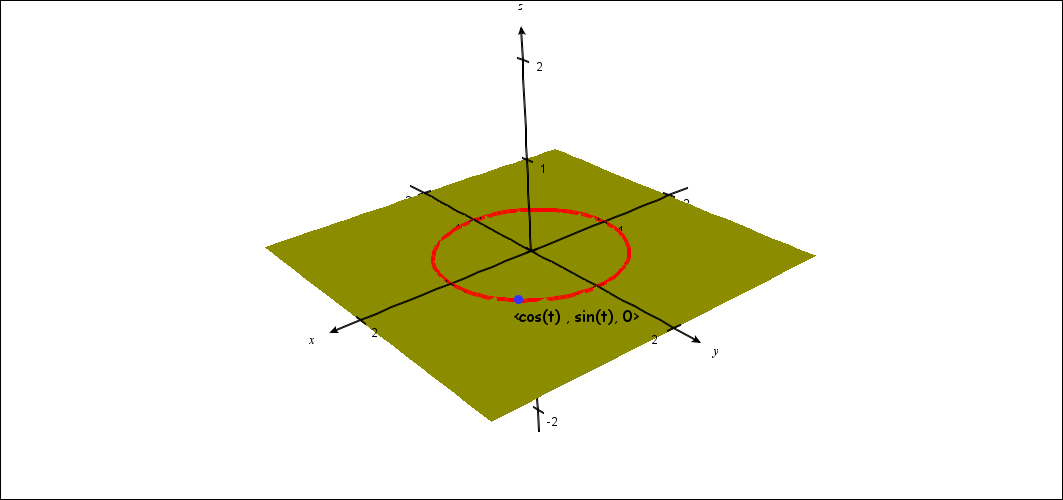# The Real Projective Plane $RP^2$

• Consider $R^3 = \{ (x,y,z) : x,y,z \in R\}$ as a vector space over $R$.
• Definition:  $v, w \in R^3 - {(0,0,0)}$ , $v$ ~ $w$ iff there is $c \ne 0$ where $w = cv$.
• FACT ~ is an equivalence relation.
• Notation:  $[v]$ ~ $=\{ w: w$ ~ $v\} =$ < $x,y,z$ >  for $v =(x,y,z) \in R^3$.
• Definition: The real projective plane: $RP^2=\{[v]$~ $:v \in R^3\ -\{(0,0,0)\}$}
• N.B.  The Euclidean plane in $RP^2$: $R^2$ "=" {< $x,y,1$ >  $: x,y \in R\}$
• An ideal point in $RP^2$: < $x,y,0$ > $\in RP^2$
• The ideal points in the real projective plane: $RP^2 - R^2 =$ {< $x,y,0$ >  $: x,y \in R\}$

•  The Euclidean Plane $R^2$ of $RP^2$ in $R^3$Ideal Points of $RP^2$  in $R^3$